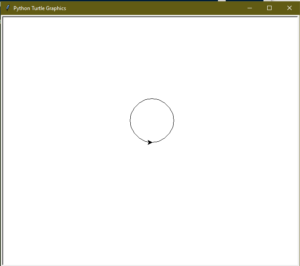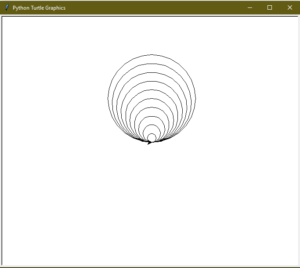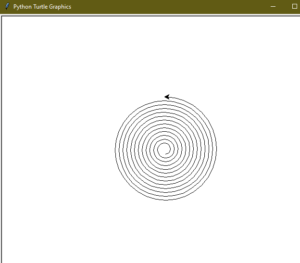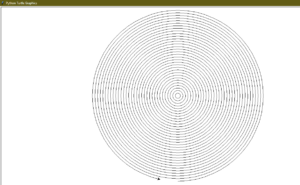Open in App
Not now

# Draw Circle in Python using Turtle

• Difficulty Level : Hard
• Last Updated : 17 May, 2022

Turtle is a Python feature like a drawing board, which lets us command a turtle to draw all over it! We can use functions like turtle.forward(…) and turtle.right(…) which can move the turtle around. Turtle is a beginner-friendly way to learn Python by running some basic commands and viewing the turtle do it graphically. It is like a drawing board that allows you to draw over it. The turtle module can be used in both object-oriented and procedure-oriented ways.
To draw, Python turtle provides many functions and methods i.e. forward, backward, etc. Some the commonly used methods are:

• forward(x): moves the pen in the forward direction by x unit.
• backward(x): moves the pen in the backward direction by x unit.
• right(x): rotate the pen in the clockwise direction by an angle x.
• left(x): rotate the pen in the anticlockwise direction by an angle x.
• penup(): stop drawing of the turtle pen.
• pendown(): start drawing of the turtle pen.

Now to draw a circle using turtle, we will use a predefined function in “turtle”.
circle(radius): This function draws a circle of the given radius by taking the “turtle” position as the center.
Example:

## Python3

 `# Python program to demonstrate ` `# circle drawing ` ` `  ` `  `import` `turtle ` `   `  `# Initializing the turtle ` `t ``=` `turtle.Turtle() ` ` `  ` `  `r ``=` `50` `t.circle(r) `

Output :#### Tangent Circles

A tangent is a line that touches the circumference of a circle from outside at a point, provided that any extension of the line will not cause intersection with the circle. Now, think about a group of circles, that have a common tangent. The group of circles, having common tangent, are known as tangent circles.
Example:

## Python3

 `# Python program to demonstrate ` `# tangent circle drawing ` ` `  ` `  `import` `turtle ` `   `  `t ``=` `turtle.Turtle() ` ` `  `# radius for smallest circle ` `r ``=` `10` ` `  `# number of circles ` `n ``=` `10` ` `  `# loop for printing tangent circles ` `for` `i ``in` `range``(``1``, n ``+` `1``, ``1``): ` `    ``t.circle(r ``*` `i) `

Output :#### Spiral Circle

Spiral is a shape similar to a circle, except that the radius of the spiral gradually increases after every completed round.
Example:

## Python3

 `# Python program to demonstrate ` `# spiral circle drawing ` ` `  ` `  `import` `turtle ` `   `  `t ``=` `turtle.Turtle() ` ` `  `# taking radius of initial radius ` `r ``=` `10` ` `  `# Loop for printing spiral circle ` `for` `i ``in` `range``(``100``): ` `    ``t.circle(r ``+` `i, ``45``) `

Output :#### Cocentric Circles

The term concentric is used for a group of things having common. Now Circles having the same center are termed as Concentric Circle.

## Python3

 `# Python program to demonstrate ` `# concentric circle drawing ` ` `  ` `  `import` `turtle ` `   `  `     `  `t ``=` `turtle.Turtle() ` ` `  `# radius of the circle ` `r ``=` `10` ` `  `# Loop for printing concentric circles ` `for` `i ``in` `range``(``50``): ` `    ``t.circle(r ``*` `i) ` `    ``t.up() ` `    ``t.sety((r ``*` `i)``*``(``-``1``)) ` `    ``t.down() `

Output :My Personal Notes arrow_drop_up
Related Articles